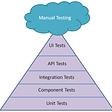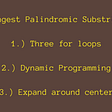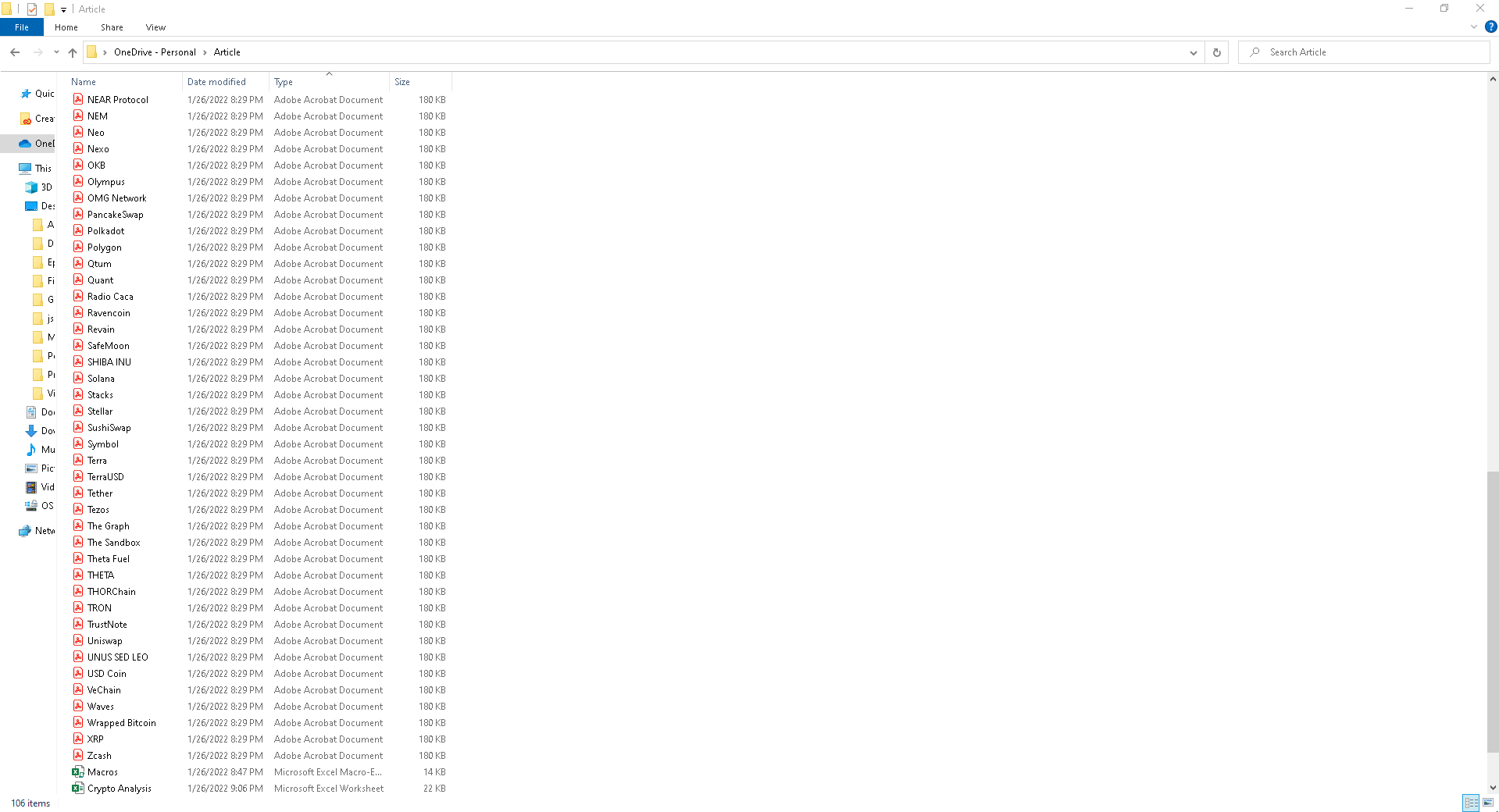# USING PYTHON GENERATE PRIME NUMBERS AND SET OPERATIONS!!!

|ODD PRIME NUMBERS||SET OPERATIONS|

In this program, we going to generate a set of prime numbers and another set of odd numbers and displaying the result of set operations.

• First, we are using the variable ”odd”. If we use a set bracket the answer will be displayed in a set bracket({}).
• And inside that set bracket, we have given list terms ”x*2+1”, this is the logic this means that we going to generate odd numbers; And we have given ”for x in range(0,5)”, which means it takes a value from “0 to 4” the odd numbers will be generated.

The logic of “x*2+1” →if value of x=1

→1*2+1

→3 ,so here we get “odd numbers”

~like that the odd numbers will be generated.

• Next, we are going to create an empty set to store prime numbers” primes =set()”; And then we are generating a for a loop.” for i in range(2,10):” which means it takes value from “2 to 9” because we are generating values up to “10”.
• Now we are going to see the prime number concept, we are going to take two variables i and j and we have to check “i/j “if we get remainder “0” it is not a prime number; “f=0” given inside the loop will act as “flag”.

# FLAG:

A flag in Python acts as a signal to the program to determine whether or not the program as a whole or a specific section of the program should run. In other words, you can set the flag to True and the program will run continuously until any type of event makes it False.

Next, “while j≤i/2:” →to check prime number

Then “if i%j==0:” →selection statement

So, we give the value of i=2 and j=2:

→2/2=0

~Remainder is “0”; likewise we can check for all the numbers.

• Then, “f=1” is not a prime number.

”j+=1”

→”if f ==0:”

• Here, if f value is “1” it will not go inside “if statement”; If f value is “0” it checks with “if statement”, then it will add prime number “primes.add(i)”.

~Like this it will repeat the process till ”9”

• Then, we going to print odd numbers, prime numbers, union, intersection, difference, symmetric difference.

--

--

--

## More from Gomathi Keerthana.R.S.

Love podcasts or audiobooks? Learn on the go with our new app.

## Good Pyramid — Bad Pyramid## Hello Medium## why Laravel’s eloquent sucks## Automation Testing Part 1: Where Do I Start?## Automated Firestore Replication to BigQuery## Popular and most-used HTML Attributes## Interview Question — All Possible Solutions## How to overcome the fear of Competitive Programming## 7 popular data visualization libraries by Python in 2021## Link Multiple Files in Excel